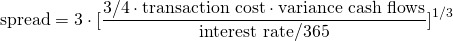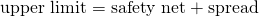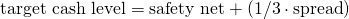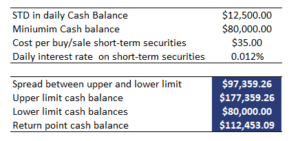# Miller-Orr model

The Miller-Orr model model is used in management to make sure a company maintains an appropriate level of cash. While too much cash is expensive because of the opportunity cost of holding cash (interest can be earned by buying short-term securities), having too little cash is risky.

Thus, CFOs should be able to determine a minimum and maximum cash balance. If the amount of cash drops below this minimum, the company should sell short-term securities. Similarly, excess cash should be invested in short-term securities to earn additional income.

On this page, we define the Miller and Orr model of cash management, discuss the Miller-Orr formula and finally conclude with a Miller-Orr model example. The spreadsheet used to create the example can be downloaded at the bottom of the page.

## Miller-Orr model formula

Let’s start by looking at the Miller-Orr model derivation. There are a number of statistics we need to calculate. First, we need to calculate the spreadwhere the transaction cost is the average cost from buying and selling short-term securities, variance of the cash flow is the volatility (in currency) of the cash flows, and the interest rate is the annual interest rate. Once we have the spread, we can use the minimum cash limit, called the safety net, and add the spread to calculate the upper limit (h)Finally, we have the target cash level (Z)## Miller-Orr calculator example

Let’s implement the above formulas and apply them to a numerical example. The following table reports the results of the exercise.The Excel file used to create the example can be downloaded at the bottom of the page.

## Summary

We discussed a very popular model of cash management. Using the formulas discussed above, treasury managers can manage the amount of cash in a company by selling short-term securities when cash is too low and buying securities when cash is too high.